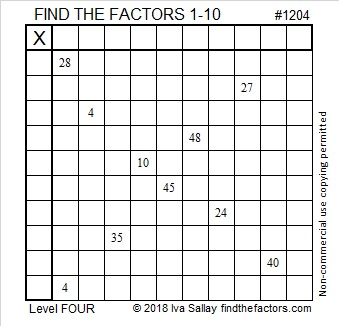# 1204 and Level 4

Today’s puzzle looks like a giant times table with a big X in the middle. The factors for this times table are not in the usual places. Can you figure out where they all go?Print the puzzles or type the solution in this excel file: 10-factors-1199-1210

Here are a few facts about the number 1204:

• 1204 is a composite number.
• Prime factorization: 1204 = 2 × 2 × 7 × 43, which can be written 1204 = 2² × 7 × 43
• The exponents in the prime factorization are 2, 1, and 1. Adding one to each and multiplying we get (2 + 1)(1 + 1)(1 + 1) = 3 × 2 × 2 = 12. Therefore 1204 has exactly 12 factors.
• Factors of 1204: 1, 2, 4, 7, 14, 28, 43, 86, 172, 301, 602, 1204
• Factor pairs: 1204 = 1 × 1204, 2 × 602, 4 × 301, 7 × 172, 14 × 86, or 28 × 43
• Taking the factor pair with the largest square number factor, we get √1204 = (√4)(√301) = 2√301 ≈ 34.69871204 is the difference of two squares two different ways:
302² – 300² = 1204
50² – 36² = 1204

This site uses Akismet to reduce spam. Learn how your comment data is processed.